## Error message

Deprecated function: The each() function is deprecated. This message will be suppressed on further calls in menu_set_active_trail() (line 2404 of /homepages/3/d408889334/htdocs/sg2/includes/menu.inc).

# Catalan Solids

The Catalan Solids are the duals of the Archimedean Solids. The vertices of the dual of a solid are the midpoints of the faces of the original solid.

Catalan Solids have nothing to do with Calalunya, they are named after their first description by belgian mathematician Eugène Catalan in 1865. The faces of these solids are not regular polygons, but they are all equal.

The following table summarizes their characteristics. It shows each Catalan Solid associated with its dual Archimedean Solid. If you click on any image, you are directed to a 3D model that you can zoom and rotate.

## The Catalan Solids and their dual Archimedean Solids

TRIAKIS TETRAHEDRON

F = 12

V =  8

E = 18TRUNCATED TETRAHEDRON

V = 12

F = 8 (4 Triangles + 4 Hexagons)

E = 18

TRIAKIS OCTAHEDRON

F = 24

V = 14

E = 38TRUNCATED CUBE

V = 24

F = 14 (8 Triangles + 6 Octagons)

E = 38

TETRAKIS HEXAHEDRON

F = 24

V =  14

E = 36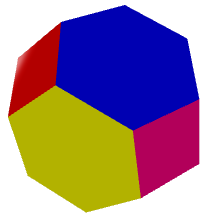TRUNCATED OCTAHEDRON

V = 24

F = 14 (8 Hexagons + 6 Squares)

E = 36

TRIAKIS ICOSAHEDRON

F = 60

V =  32

E = 90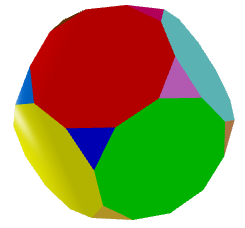TRUNCATED DODECAHEDRON

V = 60

F = 32 (20 Triangles + 12 Decagons)

E = 90

PENTAKIS DODECAHEDRON

F = 60

V =  32

E = 90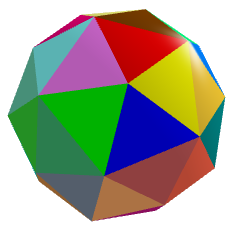TRUNCATED ICOSAHEDRON

V = 60

F = 32 (12 Pentagons + 20 Hexagons)

E = 90

RHOMBIC DODECAHEDRON

F = 12

V =  14

E = 24CUBOCTAHEDRON

V = 12

F = 14 (8 Triangles + 6 Squares)

E = 24

DISDYAKIS DODECAHEDRON

F = 48

V =  26

E = 72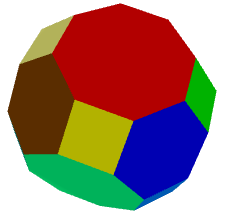TRUNCATED CUBOCTAHEDRON

V = 48

F = 26 (8 Hexa. + 12 Squa. + 6 Octa.)

E = 72

RHOMBIC TRIACONTAHEDRON

F = 30

V =  32

E = 60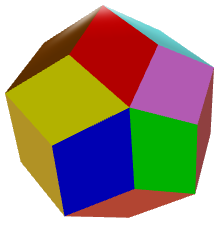ICOSIDODECAHEDRON

V = 30

F = 32 (20 Triangles + 12 Pentagons)

E = 60

DELTOIDAL ICOSITETRAHEDRON

F = 24

V =  26

E = 48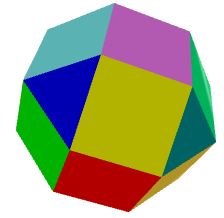RHOMBICUBOCTAHEDRON

V = 24

F = 26 (8 Triangles + 18 Squares)

E = 48

PENTAGONAL ICOSITETRAHEDRON

F = 24

V = 38

E = 60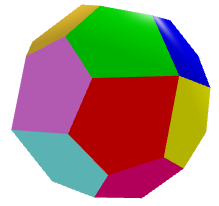SNUB CUBE

V = 24

F = 38 (32 Triangles + 6 Squares)

E = 60

DELTOIDAL HEXECONTAHEDRON

F = 60

V = 62

E = 120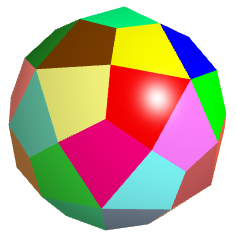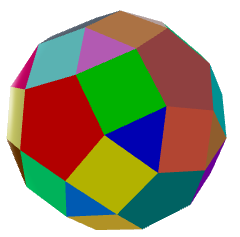RHOMBICOSIDODECAHEDRON

V = 60

F = 62 (20 Tria. + 30 Squa. + 12 Pent.)

E = 120

PENTAGONAL HEXECONTAHEDRON

F = 60

V = 92

E = 150SNUB DODECAHEDRON

V = 60

F = 92 (80 Triangles + 12 Pentagons)

E = 150

DISDYAKIS TRIACONTAHEDRON

F = 120

V = 62

E = 180TRUNCATED ICOSIDODECAHEDRON

V = 120

F = 62 (30 Squa. + 20 Hexa. + 12 Deca.)

E = 180